CivilBay

Home  > Concrete  > Concrete Anchorage  > MC Base Plate and Anchor Bolt Design - ACI 318-11 Not Using Anchor ReinforcementToolTip :

MC BASE PLATE AND ANCHOR BOLT DESIGN

Result Summary
Base Plate Design         ratio = 0.74 OK
Anchor Bolt Design
Anchor Rod Embedment, Spacing and Edge Distance       OK
Overall         ratio = 0.97 OK
Seismic Design         Tension =   OK
Shear =   OK

Design Code Reference
Anchor bolt and base plate design based on       Code Abbreviation
ACI 318-11 Building Code Requirements for Structural Concrete and Commentary Appendix D ACI 318-11
PIP STE05121 Anchor Bolt Design Guide-2006 PIP STE05121
AISC Design Guide 1: Base Plate and Anchor Rod Design 2nd Edition AISC Design Guide 1
 Load Cases Pu or Tu   [kips] Vu   [kips] Mu   [kip-ft]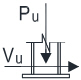LC1 Factored Axial Compression Pu = Vu =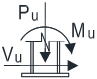LC2 Factored Axial Compression + Moment Pu = Vu = Mu =LC3 Factored Axial Compression + Moment Pu = Vu = Mu =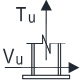LC4 Factored Axial Tensile Tu = Vu =

Base Plate & Anchor Bolt Data
Column section type   =
Column section size   =
Column section properties d = 12.100 [in] tf = 0.605 [in]
bf = 12.000 [in] tw = 0.390 [in]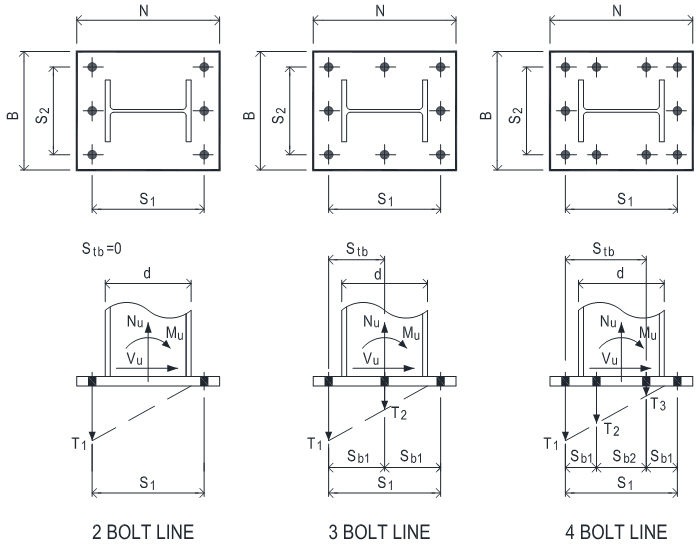Base plate width B = [in] 20.00 in
Base plate depth N = [in] 20.00 in
Base plate thickness tp = [in] 1.75 in
Suggested plate thickness for rigidity:
tp = max. of m/4  and  n/4
=

No of bolt line for resisting moment =
No of bolt along outermost bolt line =
No of bolt along side edge nbd =
PIP STE05121
Outermost bolt line spacing s1 s1 = [in] 16.00 in       Page A -1 Table 1
Outermost bolt line spacing s2 s2 = [in] 16.00 in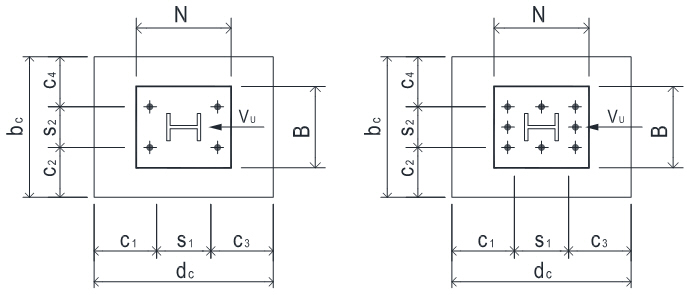PIP STE05121
Anchor bolt edge distance c1 c1 = [in] 6.00   OK   Page A -1 Table 1
Anchor bolt edge distance c2 c2 = [in] 6.00   OK
Anchor bolt edge distance c3 c3 = [in] 6.00   OK
Anchor bolt edge distance c4 c4 = [in] 6.00   OK

Anchor bolt material   =
Anchor tensile strength futa = 58.0 [ksi]
Anchor bolt diameter da  = [in] max dia=1.50 in
Anchor bolt has sleeve   =

Anchor effective cross section area Ase = 1.410 [in2]
Anchor bolt head bearing area Abrg = [in2]

Anchor bolt embedment depth hef = [in]         ACI 318-11
ci ≥ 1.5hef for at least two edges to avoid reducing of hef when Nu > 0   OK   D.6.2.3
Anchor bolt adjusted hef for design hef = [in] 18.00   OK   D.6.2.3
Pedestal height ha = [in] 21.00   OK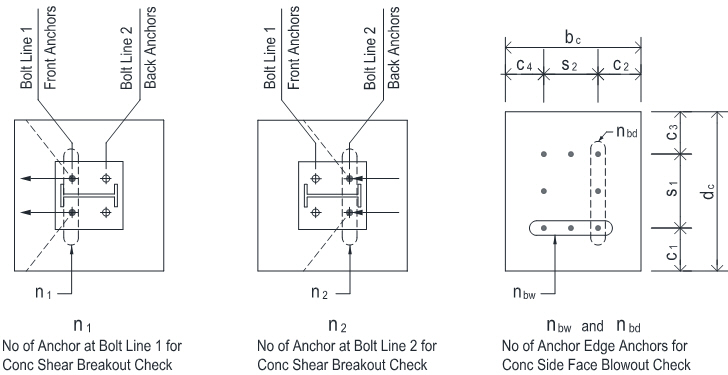For conc shear breakout check use
Number of anchor at bolt line 1 n1 =
Number of anchor at bolt line 2 n2 =

Total no of anchor bolt n =
No of anchor bolt carrying shear ns =
No of anchor bolt along width edge nbw =

Oversized holes in base plate ?   =
Concrete strength f'c = [ksi]
Base plate yield strength Fy = [ksi]
Supplementary reinforcement                 ACI 318-11
For tension   = Condition A       D.4.3 (c)
For shear Yc,v = Condition A       D.6.2.7

Concrete cracking   =         D.5.2.6, D5.3.6, D.6.2.7
Anchor bolt 1/8" (3mm) corrosion allowance =           ACI 318-11
Provide built-up grout pad ?   =           D.6.1.3

Seismic design category SDC >= C   =           D.3.3.1
Anchor bolt load E <= 0.2U Tensile =   Shear =   D.3.3.4.1 & D.3.3.5.1
Anchor bolt satisfies opion Tensile = Shear = D.3.3.4.3 & D.3.3.5.3

CONCLUSION

 LC 1 Base Plate Design Anchor Bolt DesignAnchor bolt spacing and edge distance OK ratio= 0.74 OK Overall ratio= 0.38 OK Seismic design Tension= OK Shear= OK View Detail Calc View Detail Calc LC 2 Base Plate Design Anchor Bolt DesignAnchor bolt spacing and edge distance OK ratio= 0.60 OK Overall ratio= 0.61 OK Seismic design Tension= OK Shear= OK View Detail Calc View Detail Calc LC 3 Base Plate Design Anchor Bolt DesignAnchor bolt spacing and edge distance OK ratio= 0.31 OK Overall ratio= 0.77 OK Seismic design Tension= OK Shear= OK View Detail Calc View Detail Calc LC 4 Base Plate Design Anchor Bolt DesignAnchor bolt spacing and edge distance OK ratio= 0.22 OK Overall ratio= 0.97 OK Seismic design Tension= OK Shear= OK View Detail Calc View Detail Calc

 ToolTip OnOff